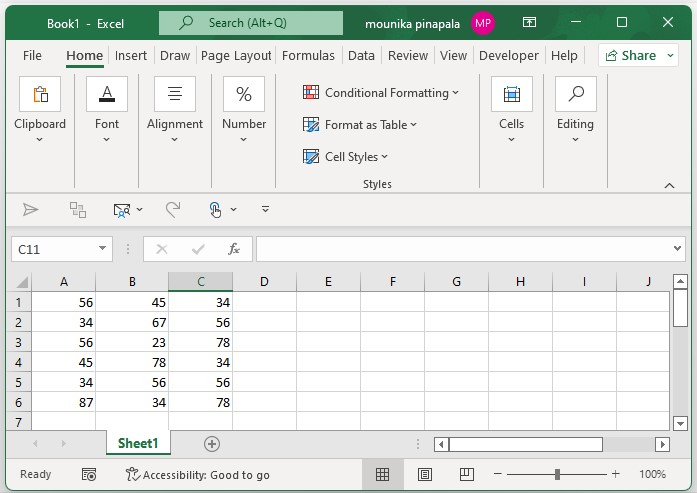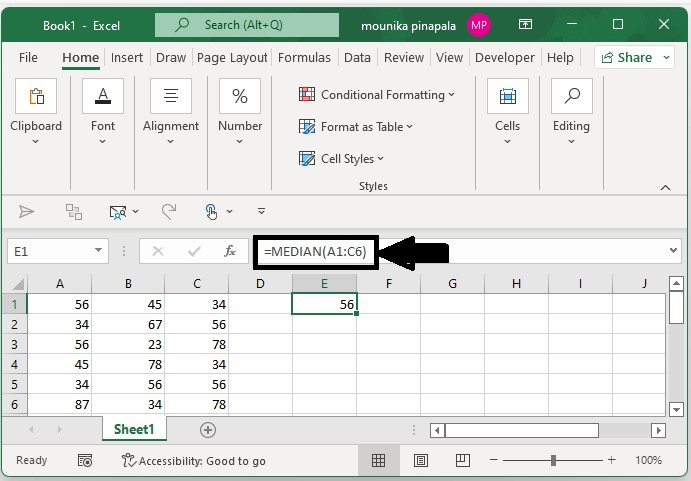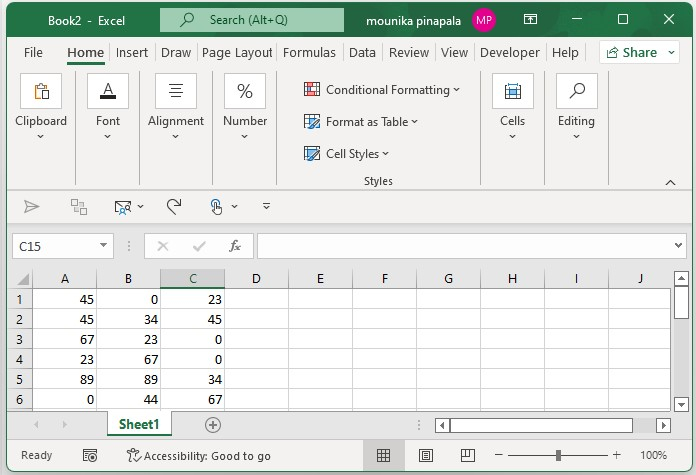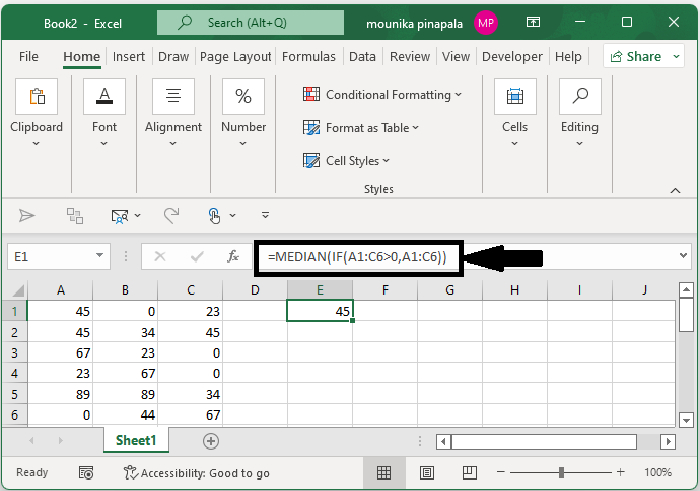# How to calculate median in a range in Excel?

Follow the steps given below to understand clearly how to calculate the median in a range in a Microsoft Excel sheet.

## Step 1

Open a Microsoft Excel sheet, and enter the data as shown in below you can also enter the data as per your wish.## Step 2

Now select a blank cell and type the below given formula in order to calculate the median and then press enter key to get the median in the cell.

=MEDIAN(A1:A6)### Note −

• In the above‑mentioned formula, A1:A6 represents the range that you want to calculate the median from).

• You can use the below given formula if the data range is not continuous.

• =MEDIAN(A1:C3,E1:G3)

• You can use the below given formula if you have the data with three discontinuous ranges.

• =MEDIAN(A1:A3,E1:G3, A5:D8)).

In the above formula, A1:C3 and E1:G3 are the ranges which you want to calculate the median from, and then the comma refers to and.

## Calculating the Median Excluding the Zero in a Range

Consider at times if the data is zero, and then you do not want to calculate the median excluding zero. In this case, you will use the below mentioned formula. Follow the steps given below −

### Step 1

Open a Microsoft excel sheet, and enter the data as shown below you can also enter the data as per your wish. Kindly find the below screenshot for your reference.### Step 2

If you do not want to calculate the median excluding zero, use the below formula. Select a blank cell enter the formula and press enter key as shown in the below screenshot.

=MEDIAN(IF(A1:C6>0,A1:C6))## Conclusion

In this tutorial, we used a simple example to demonstrate how you can calculate the median in a range in Excel.

Updated on: 03-Feb-2023

180 Views